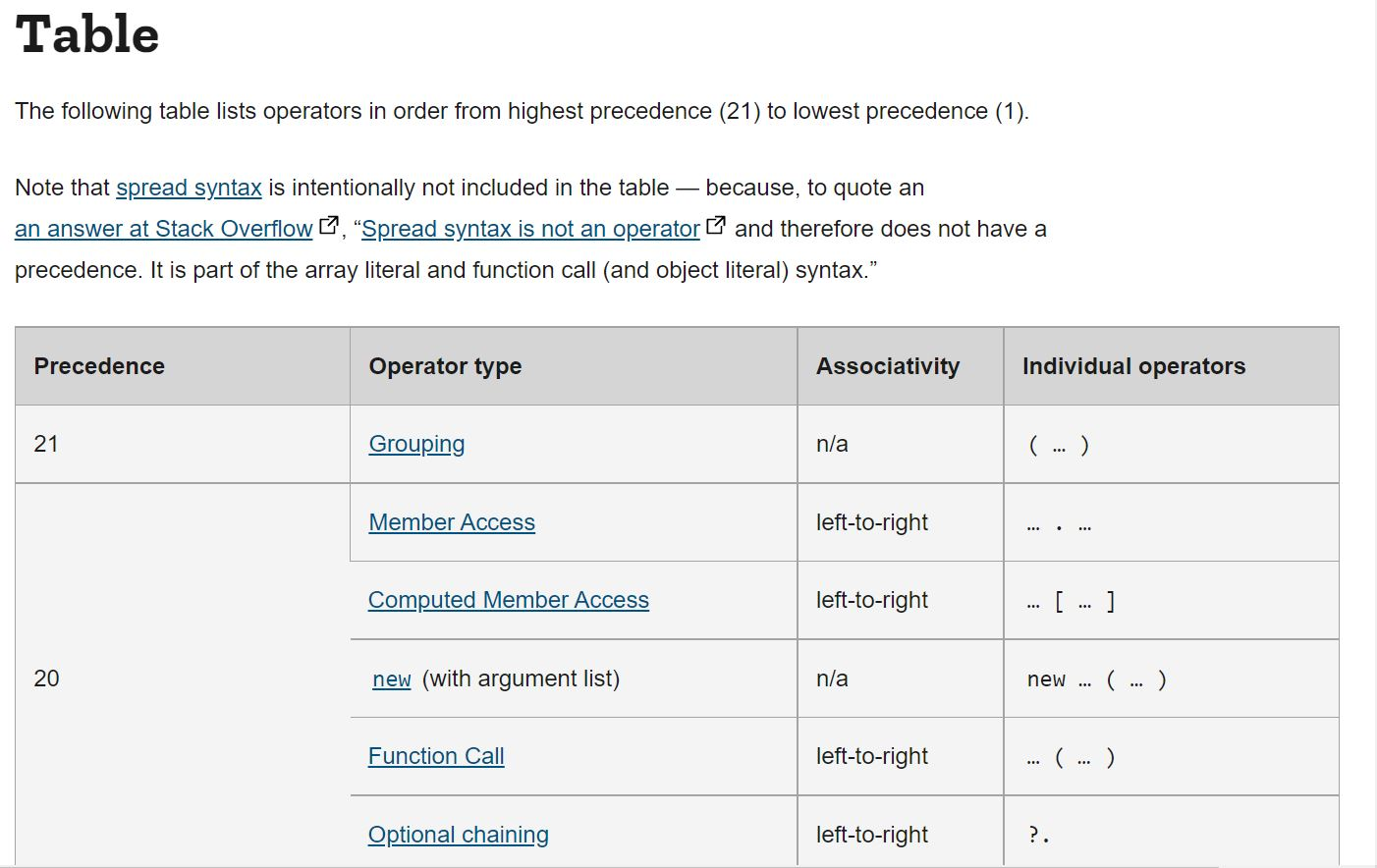# const - JS | lectureJavaScript Fundamentals 1

Operator Precedence

What is operator precedence ? How does it actually work ? Before answering theses questions, let's consider the below code snippet

script.js
``````const totalApples = 80;
const totalBananas = 90;
const remainingApples = totalApples - 25;
const remainingBananas = totalBananas - 30;
console.log(remainingApples, remainingBananas); // Result ==> 55 60
console.log(totalApples - 25 > totalBananas - 30); // Result ==> false``````

If you try to execute the above code snippet, you will notice the result of the highlighted line is false because the result of `totalApples - 25` is `55`, which is less than the result given by `totalBananas - 30` which is `60`. Now, the question is, why are those two operations first evaluated before the comparison operator ( `>` )? In short, why does it work this way? Because it actually works as we can see.

Let's try to answer all these questions. The above code snippet works the way it does because JavaScript has a well-defined order of operator precedence. That is the order in which operations are executed. To see the precedence of all operators, you can check the precedence table provided by MDNFrom the above image, you will notice, for example, that grouping ( parenthesis ) has the highest precedence of `21`, and other operations like plus ( + ), minus ( - ), and `typeof` that we used before have a precedence of `17`.

Back to the above code snippet, let's now understand how it works and what exactly happens in terms of operator precedence.

We already know that `totalApples - 25` and `totalBananas - 30` are evaluated before the comparison operator ( `>` ). The table shows that the greater than operator ( > ) has a lower precedence of `12` than the subtraction operator, which has a precedence of `14`.

You don't have to know all these numbers because no one knows. All you need is just a general idea of which operators are executed first. Usually, all mathematical operators are executed before the comparison operators.

With the table, we can also see which operators are executed from left-to-right and which one from right-to-left. We can notice, for example, from the table that the exponentiation operator is executed from right-to-left, while most of the mathematical operators are executed from left-to-right.

For example, let's have the following mathematical operation :

script.js
``````/**
* Execution is done from left to right
**/
console.log(8 * 4 / 4 + 1); // Result ==> 9``````

Let's now see an example of a right-to-left operation using the assignment operator. Consider the below code snippet :

script.js
``````let i,j; // You can define two variables on one line
i = j = 8 * 4 / 4 + 1console.log(i,j); // Result ==> i and j are both equal to 9``````

If you try to execute the above code, you will notice that `i` and `j` have a value of 9. Let's try to analyze why this happens.

When JavaScript first finds the highlighted line to execute, it will look at all the operators that are present. It will then see the mathematical operators and start with them since they have greater precedence ( Multiplication and Division: 15, Addition: 14 ) than the assignment operator (3).

After the mathematical operation, we will end up with something like this :

``i = j = 9;``

We are now left with two assignment operators to be executed, but as you can see on the table, they are executed from right-to-left. What happens is that we will have `j = 9` and finally `i = 9`. In the end, `i` and `j` are both equal to 9.

To finish this lecture, let's look at the operator with the highest precedence, grouping ( parenthesis ). Grouping works like in mathematics, where operations within parenthesis are executed first. Consider le below code snippet :

script.js
``const averageRemainingFruits = remainingApples + remainingBananas / 2console.log(averageRemainingFruits); // Result ==> 85``

Let's analyze this. According to the precedence table, we have the division operator ( 15 ) before the addition operator ( 14 ). So if we try to execute it the way it is, `remainingBananas` will be divided by 2, and the result will be added to `remainingApples`. We will then have `85` as the result.

This doesn't make sense because the average can't be greater than `remainingBananas` and `remainingApples`. That's where the parenthesis comes in. By using parenthesis, just like in mathematics, we can make that `remainingApples + remainingBananas` should be executed first since parenthesis has the highest precedence.

By adding those parenthesis, `remainingApples + remainingBananas` will be executed first, then the result will be divided by 2. By executing it now, we have the expected result which is `57.5`

script.js
``const averageRemainingFruits = (remainingApples + remainingBananas) / 2console.log(averageRemainingFruits); // Result ==> 57.5``